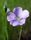## proc sql left join vs proc sql inner join

If a LEFT JOIN and an INNER JOIN return the exact same rows, which is more efficient to use?

For example,

``````data one;
mrn='1111';cpt='aaa';output;
mrn='2222';cpt='bbb';output;
mrn='3333';cpt='ccc';output;
mrn='4444';cpt='ddd';output;
run;

data two;
mrn='1111';age=22;output;
mrn='2222';age=44;output;
mrn='3333';age=66;output;
mrn='4444';age=11;output;
run;

proc sql noprint;
create table blah1 as
select
a.mrn,
a.cpt,
b.age
from
one a
inner join two b
on a.mrn=b.mrn;

create table blah2 as
select
a.mrn,
a.cpt,
b.age
from
one a
left join two b
on a.mrn=b.mrn;
quit;``````

1 ACCEPTED SOLUTION

Accepted SolutionsLinusH
Tourmaline | Level 20

## Re: proc sql left join vs proc sql inner join

To extend @Reeza answer - if your logic holds for inner joins - those are better performing than outer joins generally speaking.
Data never sleeps
3 REPLIES 3

## Re: proc sql left join vs proc sql inner join

Use the one that makes logical sense. In this case you have a 1 to 1 match. If you always expect this use an inner match, if you can't trust this rule and always want the information from a specific table use the right/left join instead.LinusH
Tourmaline | Level 20

## Re: proc sql left join vs proc sql inner join

To extend @Reeza answer - if your logic holds for inner joins - those are better performing than outer joins generally speaking.
Data never sleeps

## Re: proc sql left join vs proc sql inner join

Thanks.  I always thought INNER JOINS were not as efficient as a LEFT JOIN or a RIGHT JOIN because INNER JOINS check rows common to both tables, whereas LEFT JOINS or RIGHT JOINS just check for rows on either the left-hand or right-hand table.

I'm curious what the exceptions are to this rule, if any?

Discussion stats
• 3 replies
• 2131 views
• 0 likes
• 3 in conversation